Home / Blog / Data Science / Comparison GridSearchCV and RandomSearch CV

# Comparison GridSearchCV and RandomSearch CV

• August 04, 2023
• 2680
• 79### Meet the Author : Mr. Bharani Kumar

Bharani Kumar Depuru is a well known IT personality from Hyderabad. He is the Founder and Director of Innodatatics Pvt Ltd and 360DigiTMG. Bharani Kumar is an IIT and ISB alumni with more than 17 years of experience, he held prominent positions in the IT elites like HSBC, ITC Infotech, Infosys, and Deloitte. He is a prevalent IT consultant specializing in Industrial Revolution 4.0 implementation, Data Analytics practice setup, Artificial Intelligence, Big Data Analytics, Industrial IoT, Business Intelligence and Business Management. Bharani Kumar is also the chief trainer at 360DigiTMG with more than Ten years of experience and has been making the IT transition journey easy for his students. 360DigiTMG is at the forefront of delivering quality education, thereby bridging the gap between academia and industry.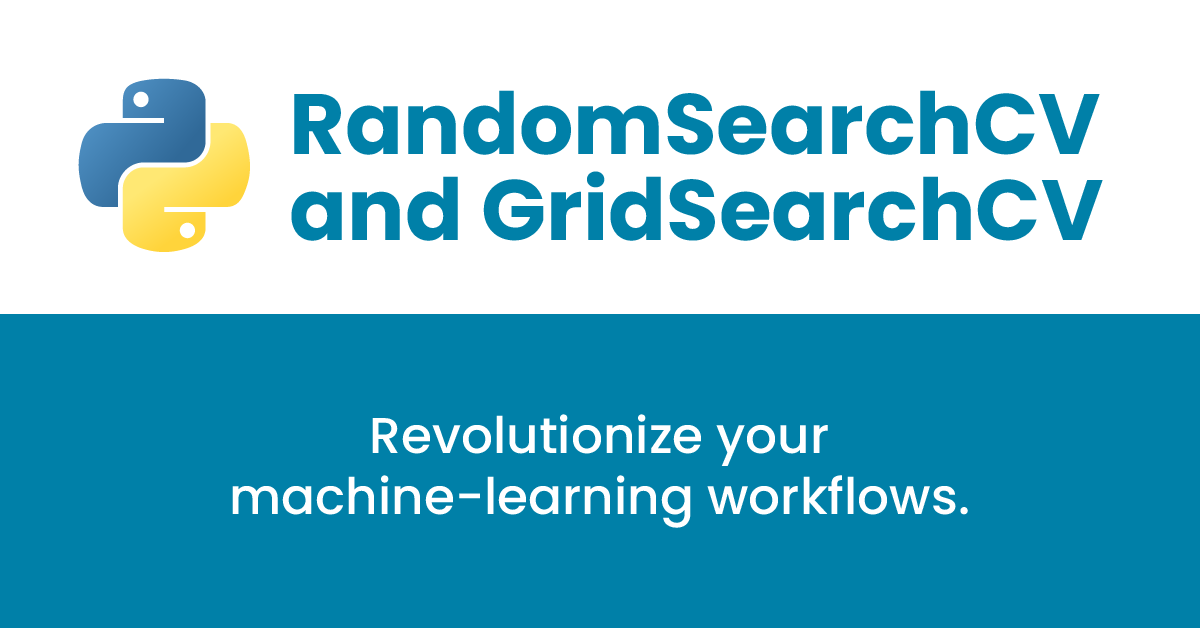## Introduction

Ever wondered how some data scientists effortlessly achieve impressive model performance while others struggle to find the right combination of hyperparameters? The secret lies in intelligent hyperparameter tuning! Brace yourself as we unveil the intriguing showdown between RandomSearchCV and GridSearchCV, paving the way to revolutionize your machine-learning workflows.

## What is Cross-validation?

Cross-validation is a crucial tool for data scientists and machine learning practitioners. It is a statistical method used to evaluate how well a machine learning model can generalize to new, unseen data. The goal of cross-validation is to estimate the performance of a model on an independent dataset, as opposed to just evaluating the model on the training dataset. In this blog, we will discuss the concept of cross-validation, its importance in machine learning, and how it works.

The term "cross-validation" refers to a set of techniques used to assess the performance of a predictive model. The fundamental concept is to split data into two or more subsets, with one subset being used to train the model and the other subset being used for testing the model's accuracy. K-fold cross-validation is the most typical kind of cross-validation. The data is randomly partitioned into k-equal subgroups, or "folds," for k-fold cross-validation. The model is tested on the last fold after being tested on k-1 folds. This process is repeated k times so that each fold is used as a testing set once. The results from each fold are then averaged to produce an overall performance estimate.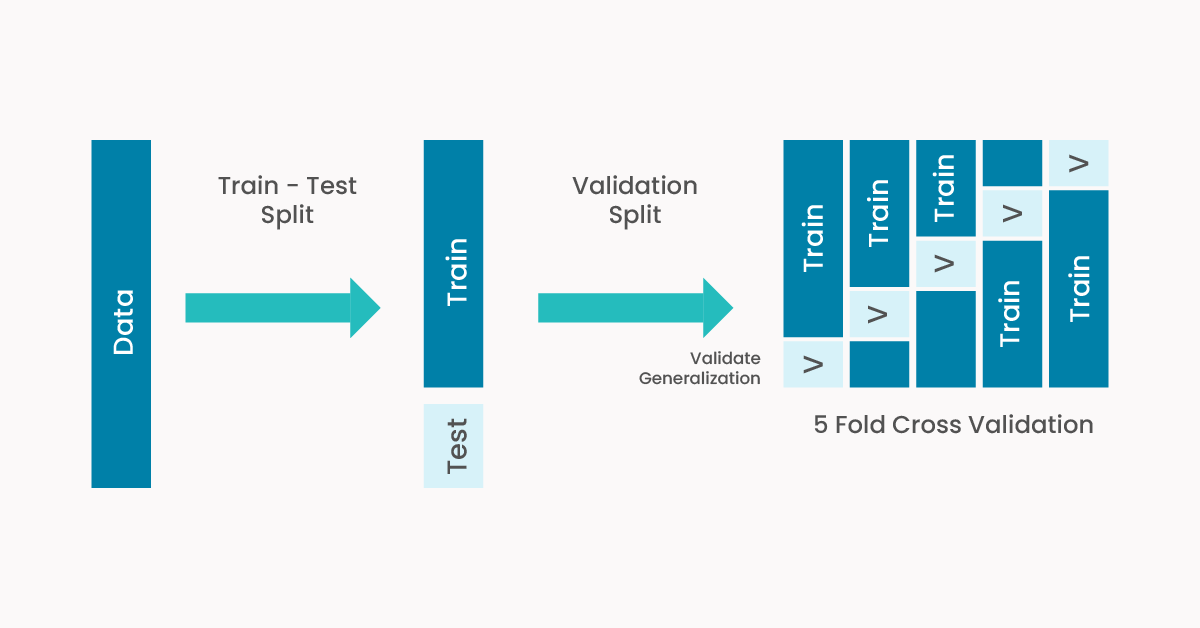Becoming a Python expert is possible now with the 360DigiTMG Python Training in Pune . Enroll today.

## Grid Search Cross-Validation

Grid search cross-validation is a technique that searches for the optimal hyperparameters of a model by evaluating the model's performance on different combinations of hyperparameter values. The idea is to define a set of hyperparameters and a range of values for each hyperparameter, and then search for the optimal combination of hyperparameters that produces the best performance on a validation set. This process is called a grid search because it searches over a grid of hyperparameters.

### Learn the core concepts of Data Science Course video on YouTube:

360DigiTMG also offers the Python Course in Bangalore to start a better career. Enroll now!

The grid search cross-validation technique can be implemented using the GridSearchCV class from the scikit-learn library in Python. The GridSearchCV class takes as input a machine-learning model, a dictionary of hyperparameters, and a cross-validation strategy. The hyperparameters dictionary contains the name of each hyperparameter and a list of values to be searched. The cross-validation strategy specifies how to split the data into training and validation sets.

Grid search cross-validation (GridSearchCV) is an effective method for enhancing a machine learning model's hyperparameters. Hyperparameters are model parameters that cannot be learned from the data, such as learning rate, regularization strength, or the number of trees in a random forest. These parameters can have a significant impact on the performance of a model, and finding the optimal values for them can be a challenging task. In this blog, we will discuss the concept of grid search cross-validation and provide a code example in Python.

#### Code Example:

Let's assume we have a dataset X and corresponding labels y, and we want to use a Random Forest Classifier as our machine learning model. We'll perform a randomized search to find the best combination of hyperparameters for the classifier.

First, make sure you have Scikit-learn installed. You can install it using pip: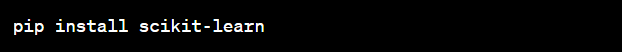Now, let's create the Python code: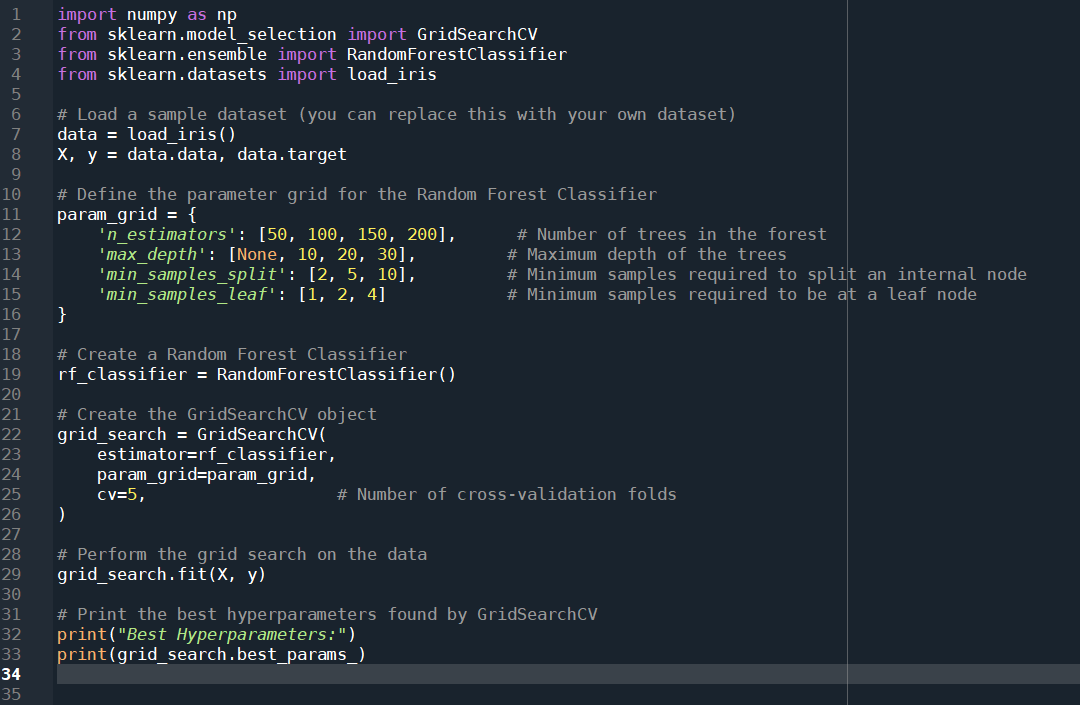Best parameters are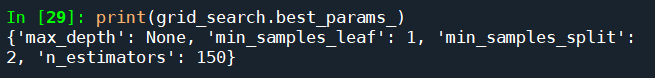The GridSearchCV will perform an exhaustive search over all the combinations of hyperparameters specified in the param_grid. It will select the best combination based on cross-validation performance.

Also, check this Python Institute in Hyderabad to start a career in Python.

## Random Search Cross-Validation

Random search cross-validation is a technique that searches for the optimal hyperparameters of a model by evaluating the model's performance on random combinations of hyperparameter values. The idea is to define a set of hyperparameters and a range of values for each hyperparameter, and then randomly sample values from these ranges to create different combinations of hyperparameters. This process is repeated a specified number of times, and the best combination of hyperparameters that produces the best performance on a validation set is selected.

The random search cross-validation technique can be implemented using the RandomizedSearchCV class from the scikit-learn library in Python. The RandomizedSearchCV class takes as input a machine learning model, a distribution of hyperparameters, and a cross-validation strategy. The distribution of hyperparameters specifies how to sample values from each hyperparameter range.

Random search cross-validation (RandomizedSearchCV) is another powerful technique for optimizing the hyperparameters of a machine learning model. It works in a similar way to grid search cross-validation, but instead of searching over a predefined grid of hyperparameters, it samples them randomly from a distribution. In this blog, we will discuss the concept of random search cross-validation and provide a code example in Python.

#### Code Example:

Let's assume we have a dataset X and corresponding labels y, and we want to use a Random Forest Classifier as our machine learning model. We'll perform a randomized search to find the best combination of hyperparameters for the classifier.

First, make sure you have Scikit-learn installed. You can install it using pip: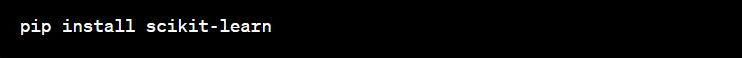Now, let's create the Python code: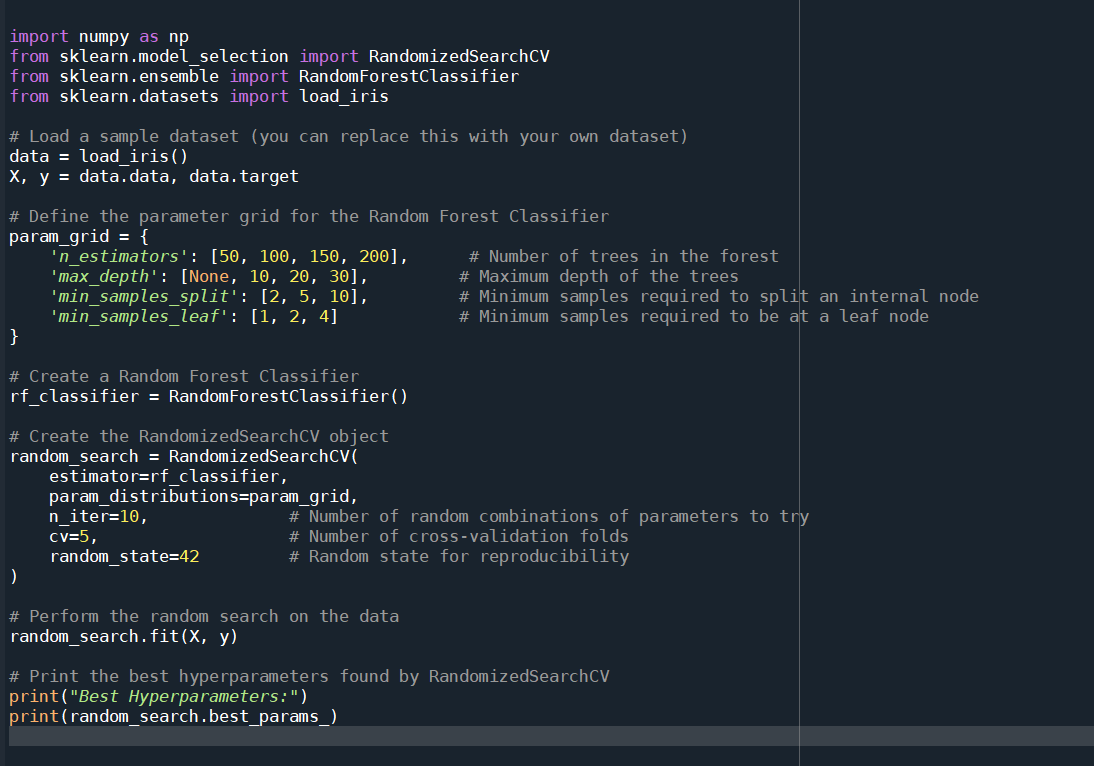Best parameters are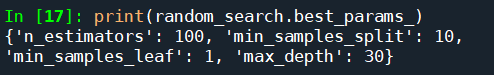The n_iter parameter controls how many random combinations of hyperparameters will be tried during the search. The number of cross-validation folds is specified by the cv option. After calling fit() with the dataset, RandomizedSearchCV will perform the search and select the best hyperparameters based on cross-validation performance. The best hyperparameters are printed at the end of the script.

## Comparison GridSearch CV and RandomSearch CV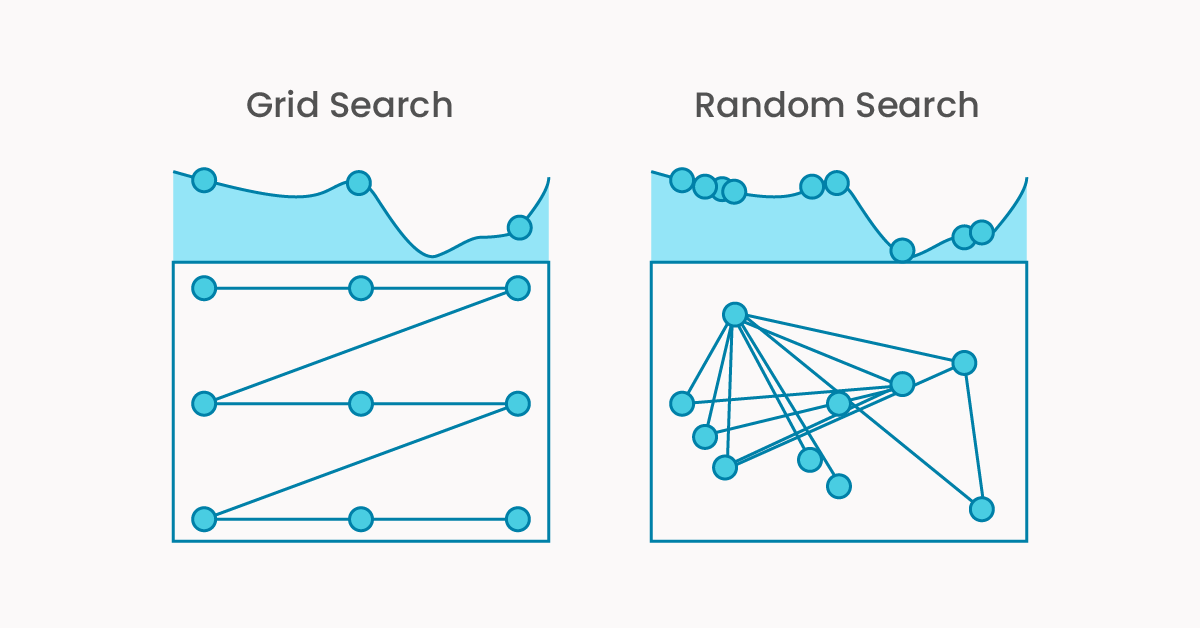Both random search and grid search cross-validation are potent techniques for optimizing the hyperparameters of a machine learning model. They work by evaluating the model's performance on different combinations of hyperparameters to find the best combination that produces the highest performance on a validation set. These two approaches, meanwhile, vary in several significant ways.

One of the main differences between random search and grid search is the way they search the hyperparameter space. Grid search evaluates the model's performance on a predefined grid of hyperparameters, whereas random search samples hyperparameters randomly from a distribution. Grid search can be more efficient in cases where the hyperparameters are highly correlated and have a strong interaction effect, but it can be computationally expensive when the hyperparameter space is large. On the other hand, the random search can be more efficient when the hyperparameter space is large and the optimal hyperparameters are not highly correlated. Another difference between random search and grid search is the number of hyperparameters they can search. Grid search can search a large number of hyperparameters, but it can become computationally expensive as the number of hyperparameters increases. Random search, on the other hand, can search a larger number of hyperparameters without becoming too computationally expensive, as it samples hyperparameters randomly.

In terms of performance, there is no clear winner between random search and grid search. It depends on the specific problem and the hyperparameter space. Random search is generally more efficient when the hyperparameter space is large and the optimal hyperparameters are not highly correlated, whereas grid search is more efficient when the hyperparameters are highly correlated and have a strong interaction effect.

Become a Python expert with 360DigiTMG Python Training in Chennai. Get trained by the 360DigiTMG.

## Conclusion:

As we conclude our exploration of hyperparameter tuning with RandomizedSearchCV and GridSearchCV, one question lingers: which path will you take? Will you embrace the dynamic and exploratory nature of RandomizedSearchCV, or opt for the exhaustive but comprehensive GridSearchCV? Share your thoughts in the comments below! Your insights and preferences matter.

### Data Analyst Courses in Other Locations